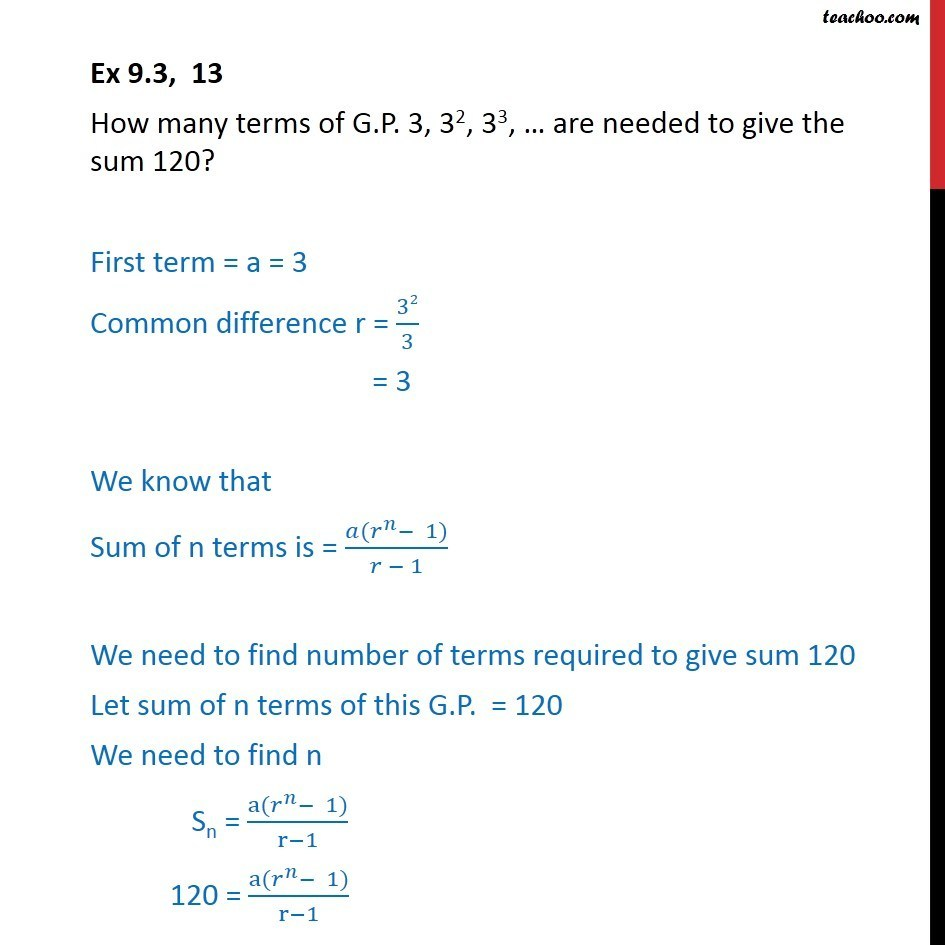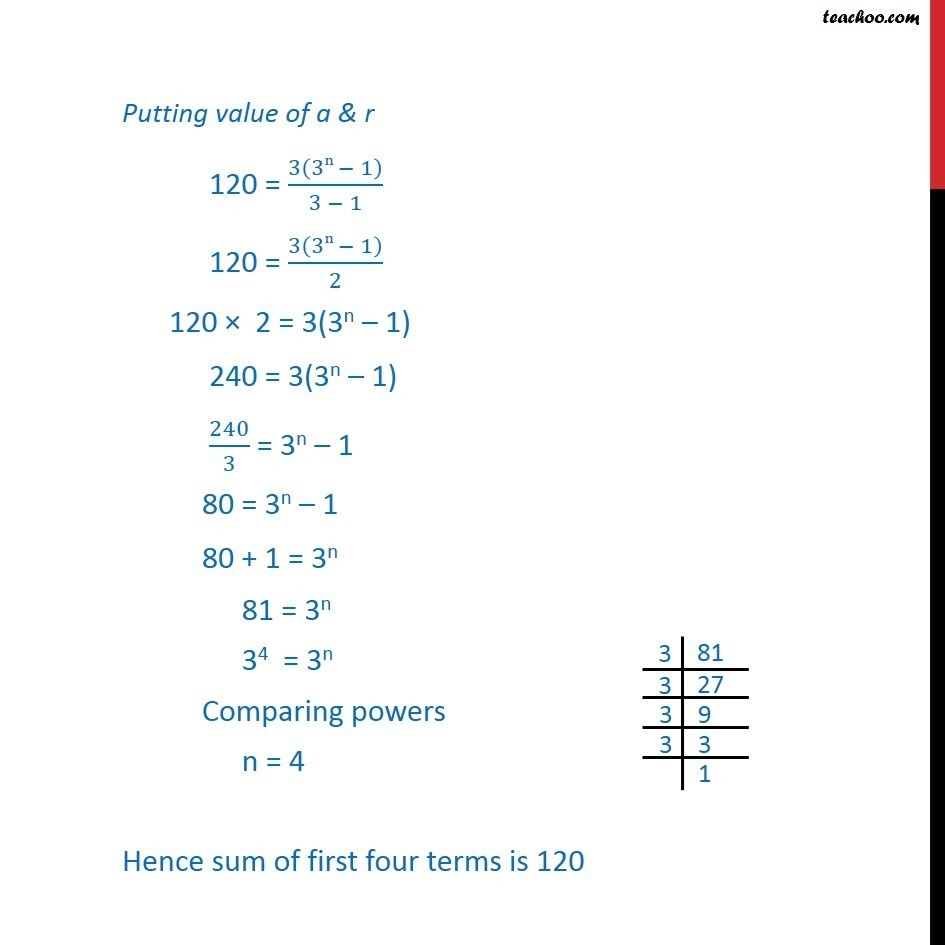Geometric Progression(GP): Formulae based

Chapter 8 Class 11 Sequences and Series
Concept wiseLearn in your speed, with individual attention - Teachoo Maths 1-on-1 Class

### Transcript

Ex 8.2, 13 How many terms of G.P. 3, 32, 33, … are needed to give the sum 120? First term = a = 3 Common difference r = 32/3 = 3 We know that Sum of n terms is = (𝑎(𝑟^𝑛− 1))/(𝑟 − 1) We need to find number of terms required to give sum 120 Let sum of n terms of this G.P. = 120 We need to find n Sn = (a(𝑟^𝑛− 1))/(r−1) 120 = (a(𝑟^𝑛− 1))/(r−1) Putting value of a & r 120 = 3(3n − 1)/(3 − 1) 120 = 3(3n − 1)/2 120 × 2 = 3(3n – 1) 240 = 3(3n – 1) 240/3 = 3n – 1 80 = 3n – 1 80 + 1 = 3n 81 = 3n 34 = 3n Comparing powers n = 4 Hence sum of first four terms is 120